Learn all Concepts of Chapter 4 Class 10 (with VIDEOS). Check - Quadratic Equations - Class 10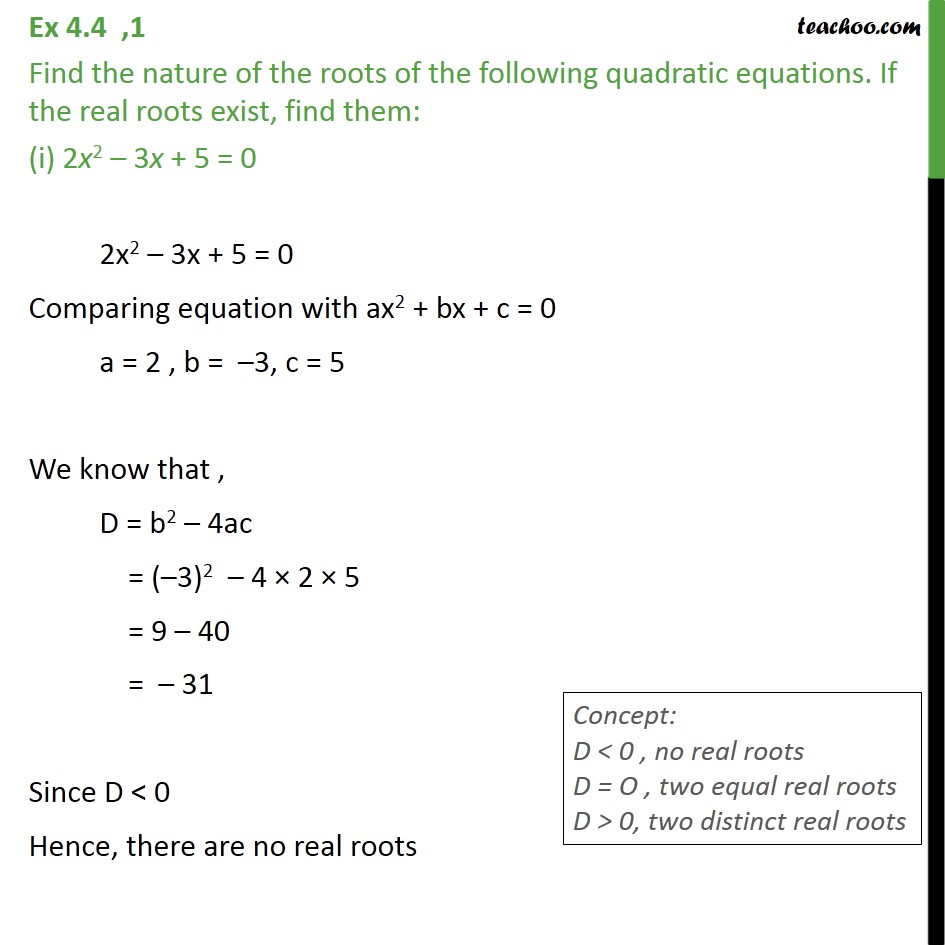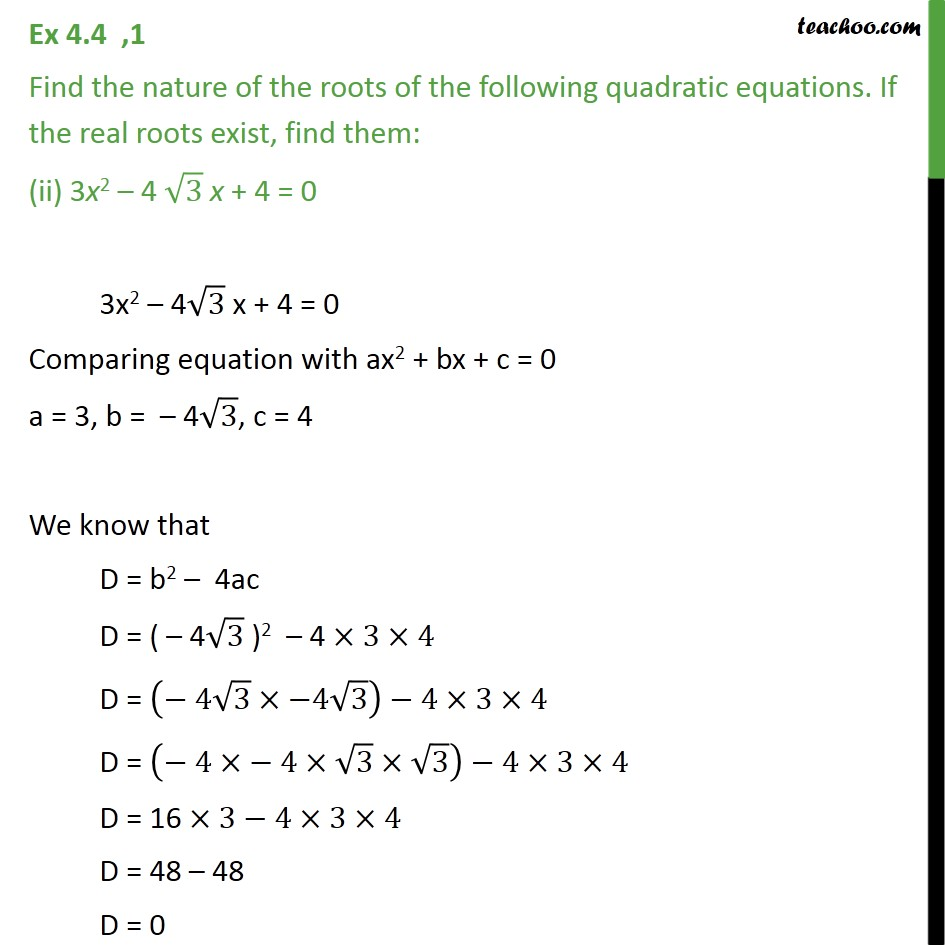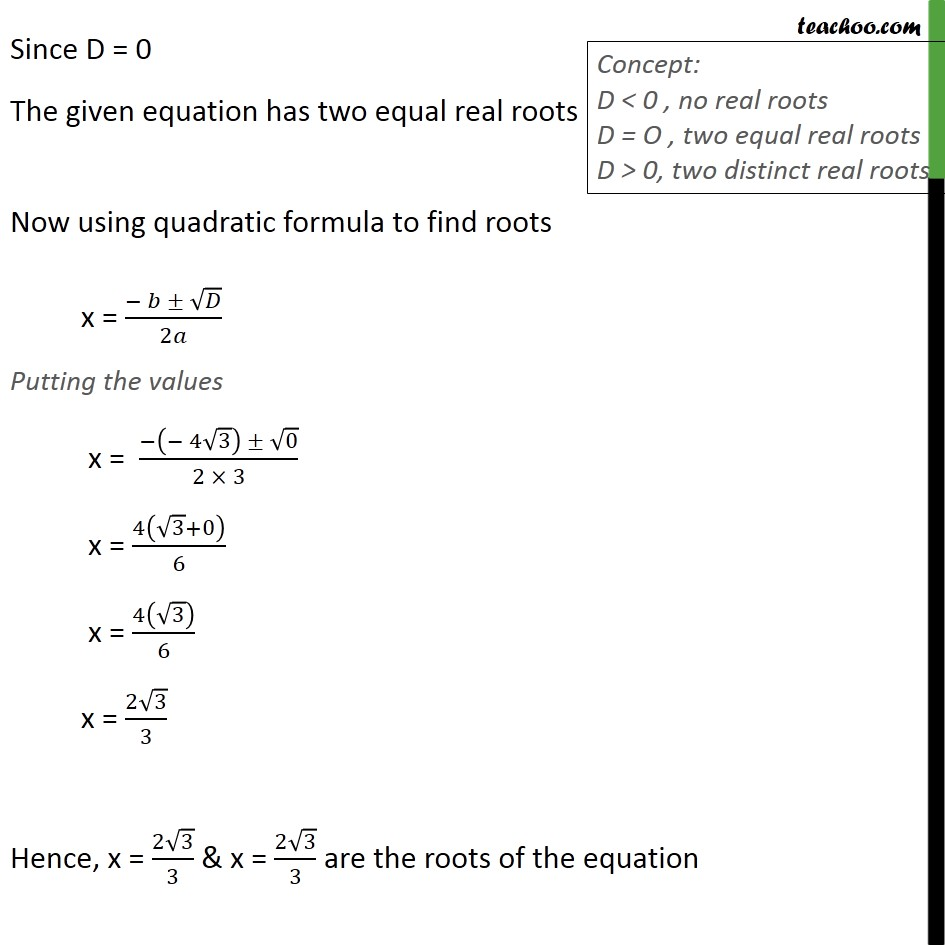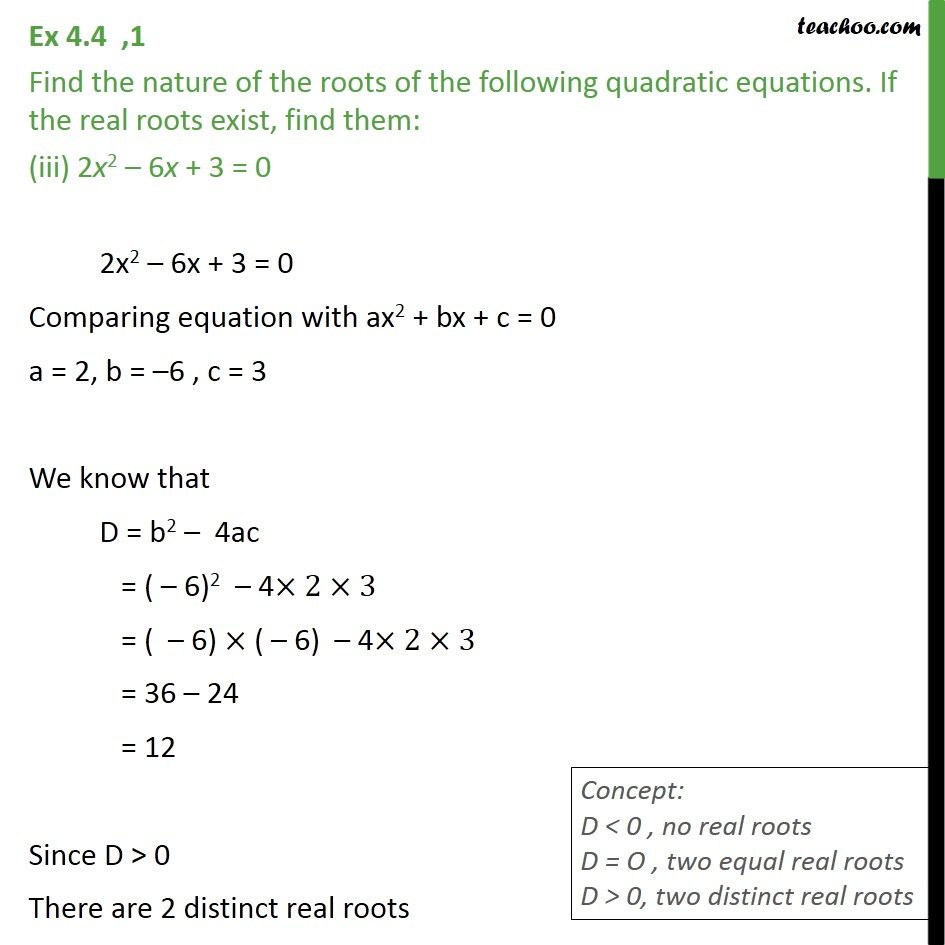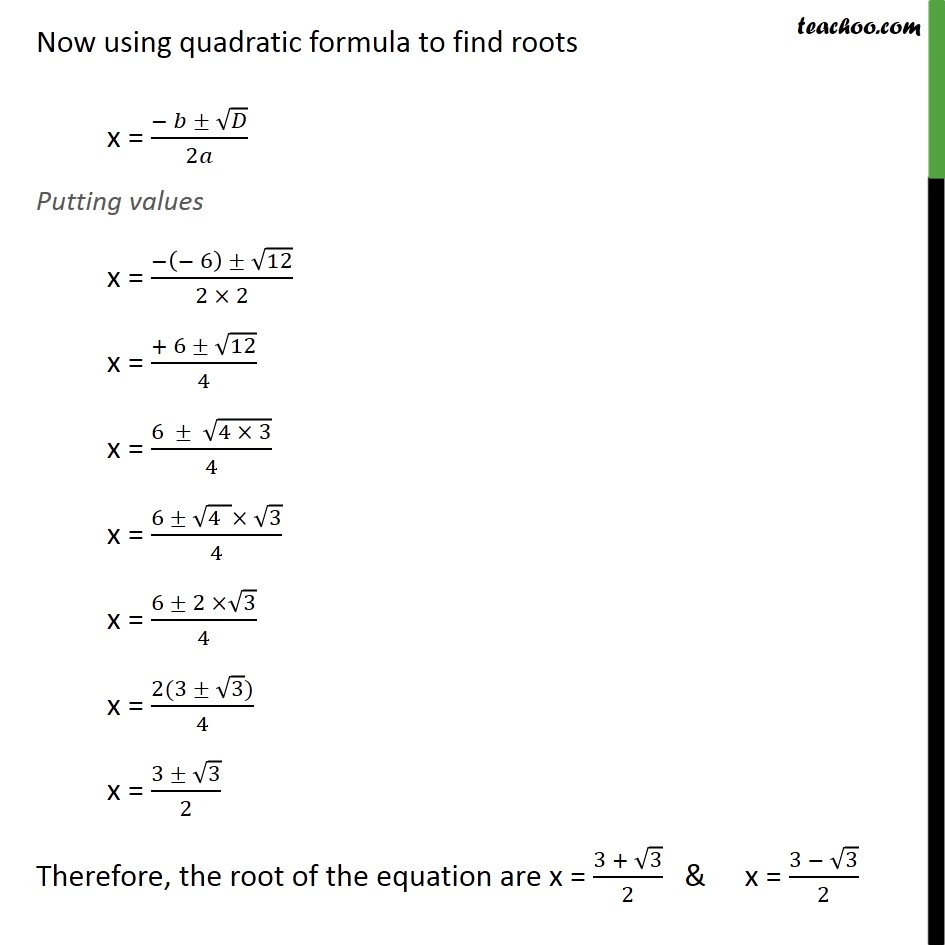1. Chapter 4 Class 10 Quadratic Equations
2. Serial order wise
3. Ex 4.4

Transcript

Ex 4.4 ,1 Find the nature of the roots of the following quadratic equations. If the real roots exist, find them: (i) 2x2 – 3x + 5 = 0 2x2 – 3x + 5 = 0 Comparing equation with ax2 + bx + c = 0 a = 2 , b = –3, c = 5 We know that , D = b2 – 4ac = (–3)2 – 4 × 2 × 5 = 9 – 40 = – 31 Since D < 0 Hence, there are no real roots Ex 4.4 ,1 Find the nature of the roots of the following quadratic equations. If the real roots exist, find them: (ii) 3x2 – 4 √3 x + 4 = 0 3x2 – 4√3 x + 4 = 0 Comparing equation with ax2 + bx + c = 0 a = 3, b = – 4√3, c = 4 We know that D = b2 – 4ac D = ( – 4√3 )2 – 4 ×3×4 D = (− 4√3×−4√3)−4×3×4 D = (− 4×− 4×√3×√3)−4×3×4 D = 16 ×3−4×3×4 D = 48 – 48 D = 0 Since D = 0 The given equation has two equal real roots Now using quadratic formula to find roots x = (− 𝑏 ± √𝐷)/2𝑎 Putting the values x = (−(− 4√3) ± √0)/(2 × 3) x = 4(√3+0)/6 x = 4(√3)/6 x = (2√3)/3 Hence, x = (2√3)/3 & x = (2√3)/3 are the roots of the equation Ex 4.4 ,1 Find the nature of the roots of the following quadratic equations. If the real roots exist, find them: (iii) 2x2 – 6x + 3 = 0 2x2 – 6x + 3 = 0 Comparing equation with ax2 + bx + c = 0 a = 2, b = –6 , c = 3 We know that D = b2 – 4ac = ( – 6)2 – 4×2×3 = ( – 6) × ( – 6) – 4×2×3 = 36 – 24 = 12 Since D > 0 There are 2 distinct real roots Now using quadratic formula to find roots x = (− 𝑏 ± √𝐷)/2𝑎 Putting values x = (−(− 6) ± √12)/(2 × 2) x = (+ 6 ± √12)/4 x = (6 ± √(4 × 3))/4 x = (6 ± √(4 )× √3)/4 x = (6 ± 2 ×√3)/4 x = (2(3 ± √3))/4 x = (3 ± √3)/2 Therefore, the root of the equation are x = (3 + √3)/2 & x = (3 − √3)/2

Ex 4.4